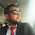## Friday, March 29, 2013

### Scala Call-By-Value Versus Call-By-Name

This tutorial demonstrates the difference between call-by-value and call-by-name method parameters. By default, method arguments are passed call-by-value. This is the evaluation that you're probably already familiar with from Java. With call-by-value, the arguments that are passed to the method are evaluated as an expression before the control flow is passed to the method. Here are two methods with the exact same code in the method body. They simply execute a loop for five iterations, referencing the parameter "x" within each iteration. The only difference is that the first method takes a call-by-value parameter (notice the => syntax), while the second method takes a call-by-name parameter.

```  def callByValue(x : Unit) = {
for (i <- 0 until 5) {
print(x)
}
}

def callByName(x : => Unit) = {
for (i <- 0 until 5) {
print(x)
}
}
```

Now, let's see what happens to the value of the parameter when we call these two methods with an expression that increments the function value by one (i = i + 1). When we call the callByValue method, the result of the value after the function call is simply i = i + 1.

```  def main(args: Array[String]) {
var i = 0

callByValue(i = i + 1)

print(i)  // "1"
}
```

What's happening here is that the expression i = i + 1 is being evaluated one time, before the control flow is passed to the callByValue method. However, after calling the callByName method instead of callByValue, the result of the variable "i" is 5.

```  def main(args: Array[String]) {
var i = 0

callByName(i = i + 1)

print(i)  // "5"
}
```

The reason this is happening is because when a parameter is call-by-name, the value of the parameter is evaluated every time it's referenced in the method. It's being referenced 5 times, so the expression i = i + 1 is being executed 5 times.

#### 1 comment:

1.nice explanaion